# 15. Missing, Censored, and Questionable Data

The online version of the open-access textbook Minimalist Data Wrangling with Python by Marek Gagolewski is, and will remain, freely available for everyone’s enjoyment (also in PDF). Any bug/typos reports/fixes are appreciated. Although available online, this is a whole course; it should be read from the beginning to the end. In particular, refer to the Preface for general introductory remarks.

So far we have been assuming that observations are of “decent quality”, i.e., trustworthy. It would be nice if in reality that was always the case, but it is not.

In this section we briefly address the most basic methods for dealing with “suspicious” observations: outliers, missing, censored, and incorrect data.

## 15.1. Missing Data

Consider the nhanes_p_demo_bmx_2020 dataset being another excerpt from the National Health and Nutrition Examination Survey by the US Centres for Disease Control and Prevention:

nhanes = pd.read_csv("https://raw.githubusercontent.com/gagolews/" +
"teaching_data/master/marek/nhanes_p_demo_bmx_2020.csv",
comment="#")
##      SEQN  BMDSTATS  BMXWT  ...  SDMVPSU  SDMVSTRA  INDFMPIR
## 0  109263         4    NaN  ...        3       156      4.66
## 1  109264         1   42.2  ...        1       155      0.83
## 2  109265         1   12.0  ...        1       157      3.06
##
## [3 rows x 50 columns]


Some of the columns feature NaN (not-a-number) values, which are used here to encode missing data.

The reasons behind why some items are missing might be numerous, for instance:

• a participant does not know the answer to a given question;

• a patient refused to answer a given question;

• a participant does not take part in the study anymore (attrition, death, etc.);

• an item is not applicable (e.g., number of minutes spent cycling weekly when someone answered they do not like bikes at all);

• a piece of information was not collected, e.g., due to the lack of funding or equipment failure.

### 15.1.1. Representing and Detecting Missing Values

Sometimes missing values will be specially encoded, especially in CSV files, e.g., with -1, 0, 9999, numpy.inf, -numpy.inf, or None, strings such as "NA", "N/A", "Not Applicable", "---" – we should always inspect our datasets carefully.

Generally, we will be converting them to NaN (as in: numpy.nan) in numeric (floating-point) columns or to Python’s None otherwise, to assure consistent representation.

Vectorised functions such as numpy.isnan (or, more generally, numpy.isfinite) and pandas.isnull as well as isna methods for the DataFrame and Series classes can be used to verify whether an item is missing or not.

For instance, here are the counts and proportions of missing values in each column (we will display only the top 5 columns to save space):

nhanes_na_stats = nhanes.isna().apply([np.sum, np.mean]).T
nhanes_na_stats.nlargest(5, "sum")
##               sum      mean
## BMIRECUM  14257.0  0.996993
## BMIHT     14129.0  0.988042
## BMIHIP    13924.0  0.973706


Looking at the column descriptions, BMIHEAD stands for “Head Circumference Comment”, whereas BMXHEAD is “Head Circumference (cm)”, but it is only applicable for infants.

Exercise 15.1

Read the column descriptions (refer to the comments in the CSV file for the relevant URLs) to identify the possible reasons for some of the NHANES data being missing.

Exercise 15.2

Study the pandas manual to learn about the difference between the DataFrameGroupBy.size and DataFrameGroupBy.count methods.

### 15.1.2. Computing with Missing Values

Our use of NaN to denote a missing piece of information is actually an ugly (but still functioning) hack. The original use case for not-a-number is to represent the results of incorrect operations, e.g., logarithms of negative numbers or subtracting two infinite entities. Therefore, we need extra care when handling them. On a side note, e.g., the R environment has a built-in, seamless support for missing values.

Generally, arithmetic operations on missing values yield a result that is undefined as well:

np.nan + 2  # "don't know" + 2 == "don't know"
## nan
np.mean([1, np.nan, 2, 3])
## nan


There are versions of certain aggregation functions that ignore missing values whatsoever: numpy.nanmean, numpy.nanmin, numpy.nanmax, numpy.nanpercentile, numpy.std, etc.

np.nanmean([1, np.nan, 2, 3])
## 2.0


However, running aggregation functions directly on Series objects ignores missing entities by default. Compare an application of numpy.mean on a Series instance vs on a vector:

x = nhanes.loc[:6, "BMXHT"]  # some example Series, whatever
np.mean(x), np.mean(x.to_numpy())
## (148.5, nan)


This is quite an unfortunate behaviour, because this way we might miss (sic!) the presence of missing values. This is why it is very important always to pre-inspect a dataset carefully.

Also, due to NaN’s being of floating-point type, it cannot be present in, amongst others, logical vectors. By convention, comparisons against missing values yield False (instead of the more semantically valid missing value):

x  # preview
## 0      NaN
## 1    154.7
## 2     89.3
## 3    160.2
## 4      NaN
## 5    156.0
## 6    182.3
## Name: BMXHT, dtype: float64
y = (x > 40)
y
## 0    False
## 1     True
## 2     True
## 3     True
## 4    False
## 5     True
## 6     True
## Name: BMXHT, dtype: bool


Note

(*) If we want to retain the missingness information (we do not know if a missing value is greater than 40), we need to do it manually:

y = y.astype("object")  # required for numpy vectors, not for pandas Series
y[np.isnan(x)] = None
y
## 0    None
## 1    True
## 2    True
## 3    True
## 4    None
## 5    True
## 6    True
## Name: BMXHT, dtype: object

Exercise 15.3

Read the pandas manual for more technical details on missing value handling.

### 15.1.3. Missing at Random or Not?

At a general level (from the mathematical modelling perspective), we may distinguish between different missingness patterns (as per Rubin’s [Rub76]):

• missing completely at random: reasons are unrelated to data and probabilities of cases being missing are all the same;

• missing at random: there are different probabilities of being missing within different groups (e.g., males might systematically refuse to answer specific questions);

• missing not at random: due to reasons unknown to us (e.g., data was collected at different times, there might be systematic differences but within the groups that we cannot easily identify, e.g., amongst participants with data science background where we did not ask about education or occupation).

It is important to try to determine the reason for missingness, because this will usually imply the kinds of techniques that are more or less suitable in specific cases.

We may try removing (discarding) the rows or columns that feature at least one, some, or too many missing values.

However, for this we need to be “rich enough” – such a scheme will obviously not work for small datasets, where each observation is precious (but on the other hand, if we want to infer from too small datasets, we should ask ourselves whether this is a good idea at all… it might be better to simply refrain from any data analysis than to come up with conclusions that are likely to be unjustified).

Also, we should not exercise data removal in situations where missingness is conditional (e.g., data only available for infants) or otherwise group-dependent (not-completely at random; e.g., it might result in an imbalanced dataset).

Exercise 15.4

With the nhanes_p_demo_bmx_2020 dataset:

1. remove all columns that are comprised of missing values only,

2. remove all columns that are made of more than 20% missing values,

3. remove all rows that only consist of missing values,

4. remove all rows that feature at least one missing value,

5. remove all columns that feature at least one missing value.

Hint: pandas.DataFrame.dropna might be useful in the simplest cases, and numpy.isnan or pandas.DataFrame.isna with loc[...] or iloc[...] otherwise.

### 15.1.5. Mean Imputation

When we cannot afford or it is inappropriate/inconvenient to proceed with the removal of missing observations or columns, we might try applying some missing value imputation techniques. Although, let us be clear – this is merely a replacement thereof by some hopefully useful guesstimates.

Important

Whatever we decide to do with the missing values, we need to be explicit about the way we have handled them in all the reports from data analysis, as sometimes they might strongly affect the results.

Let us consider an example vector with missing values, comprised of heights of the adult participants of the NHANES study.

x = nhanes.loc[nhanes.loc[:, "RIDAGEYR"] >= 18, "BMXHT"]


The simplest approach is to replace each missing value with the corresponding column’s mean (for each column separately). This does not change the overall average (but decreases the variance).

xi = x.copy()
xi[np.isnan(xi)] = np.nanmean(xi)


Similarly, we could have considered replacing missing values with the median, or – in case of categorical data – the mode.

Our height data are definitely not missing completely at random – in particular, we expect heights to differ, on average, between sexes. Therefore, another basic imputation option is to replace the missing values with the corresponding within-group averages:

xg = x.copy()
g = nhanes.loc[nhanes.loc[:, "RIDAGEYR"] >= 18, "RIAGENDR"]
xg[np.isnan(xg) & (g == 1)] = np.nanmean(xg[g == 1])  # male
xg[np.isnan(xg) & (g == 2)] = np.nanmean(xg[g == 2])  # female


Unfortunately, whichever imputation method we choose, it will artificially distort the data distribution (and hence introduce some kind of bias), see Figure 15.1.

plt.subplot(1, 3, 1)
sns.histplot(x, binwidth=1, binrange=[130, 200], color="lightgray")
plt.ylim(0, 500)
plt.title("Original")
plt.subplot(1, 3, 2)
sns.histplot(xi, binwidth=1, binrange=[130, 200], color="lightgray")
plt.ylim(0, 500)
plt.title("Replace by mean")
plt.subplot(1, 3, 3)
sns.histplot(xg, binwidth=1, binrange=[130, 200], color="lightgray")
plt.ylim(0, 500)
plt.title("Replace by group mean")
plt.show()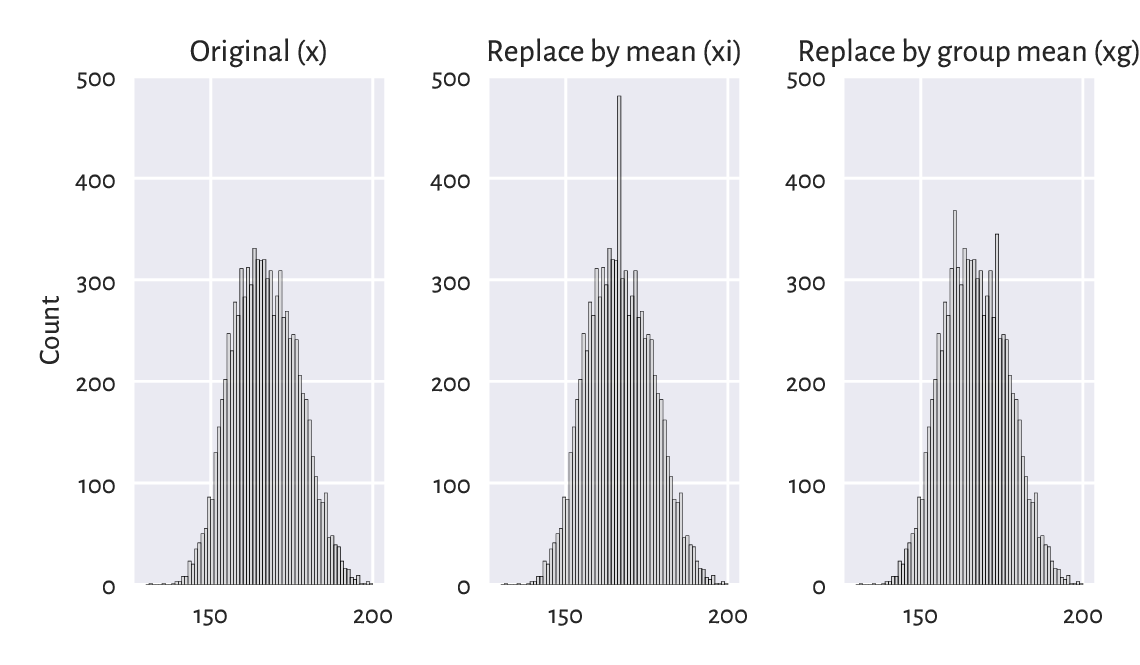Figure 15.1 Mean imputation distorts the data distribution

These effects might not be visible if we increase the bin widths, but they are still there. After all, we have added to the sample many identical values.

Exercise 15.5

With the nhanes_p_demo_bmx_2020 dataset:

1. for each numerical column, replace all missing values with the column averages,

2. for each categorical column, replace all missing values with the column modes,

3. for each numerical column, replace all missing values with the averages corresponding to a patient’s sex (as given by the RIAGENDR column).

Note

(*) We can easily implement a missing value imputer based on averaging data from an observation’s non-missing nearest neighbours, compare {numref}sec:knn. This is an extension of the simple idea of finding the most “similar” observation (with respect to chosen criteria) to a given one and “borrowing” missing measurements from it. More generally, different regression or classification models can be built on non-missing data and then the missing observations can be replaced by the values predicted by those models.

Note

(**) Rubin (e.g., [LR02]) suggests the use of a procedure called multiple imputation (see also [vB18]), where copies of the original datasets are created, missing values are imputed by sampling from some estimated distributions, inference is made, and then the results are aggregated. An example implementation of such an algorithm is available in sklearn.impute.IterativeImputer.

## 15.2. Censored and Interval Data (*)

Censored data frequently appear in the context of reliability, risk analysis, and biostatistics, where the observed objects might “fail” (e.g., break down, die, withdraw), compare, e.g., [MKK16]. Our introductory course cannot obviously cover everything, but a beginner analyst should at least be aware of such data being a thing, in particular:

• right-censored data: we only know that the actual value is above the recorded one (e.g., we stopped the experiment on the reliability of light bulbs after 1000 hours, so those which still work will not have a time-of-failure precisely known);

• left-censored data: the actual observation is below the recorded one, e.g., we observe a component’s failure, but we do not know for how long it has been in operation before the study has started.

Hence, in such cases, the recorded datum of, say, 1000, can actually mean $$[1000, \infty)$$, $$[0, 1000]$$, or $$(-\infty, 1000]$$.

There might also be instances where we know that a value is in some interval $$[a, b]$$. There are numerical libraries that deal with interval computations, and some data analysis methods exist in such a case.

## 15.3. Incorrect Data

Missing data might already be present in a given sample but also we might be willing to mark some existing values as missing, e.g., when they are simply incorrect.

For example:

• for text data, misspelled words;

• for spatial data, GPS coordinates of places out of this world, non-existing zip codes, or invalid addresses;

• for date-time data, misformatted date-time strings, incorrect dates such as “29 February 2011”, an event’s start date being after the end date;

• for physical measurements, observations that do not meet specific constraints, e.g., negative ages, or heights of people over 300 centimetres;

• IDs of entities that simply do not exist (e.g., unregistered or deleted clients’ accounts);

and so forth.

In order to be able to identify and handle incorrect data, we need specific knowledge valid for a particular domain. Optimally, basic data validation techniques should already be employed on the data collection stage, for instance when a user submits an online form.

There can be many tools that can assist us with identifying errorneous observations, e.g., spell checkers such as hunspell.

For smaller datasets, observations can also be manually inspected. However, sometimes we will have to develop our own algorithms for detecting “bugs” in data.

Exercise 15.6

Given some data frame with numeric columns only, perform what follows.

1. Check if all numeric values in each column are between 0 and 1000.

2. Check if all values in each column are unique.

3. Verify that all the rowwise sums add up to 1.0 (up to a small numeric error).

4. Check if the data frame consists of 0s and 1s only. It that is the case, verify that for each row, if there is a 1 in the first column, then all the remaining columns are filled with 1s too.

Many data validation methods can be reduced to operations on strings. They may be as simple as writing a single regular expression or checking if a label is in a dictionary of possible values and as difficult as writing your own parser for a custom context-sensitive grammar.

### 15.3.1. Exercises on Validating Data

Once we have imported the data fetched from different sources, it will usually be the case that relevant information will have to be extracted from raw text, e.g., strings like "1" should be converted to floating-point numbers. Below we suggest a number of tasks that aid in developing data validation skills involving some operations on textual information.

Exercise 15.7

Given an example data frame with text columns (manually invented, be creative), perform what follows.

1. Remove trailing and leading whitespaces from each string.

2. Check if all strings can be interpreted as numbers, e.g., "23.43".

3. Verify if a date string in YYYY-MM-DD format is correct.

4. Determine if a date-time string in YYYY-MM-DD hh:mm:ss format is correct.

5. Check if all strings are of the form (+NN) NNN-NNN-NNN or (+NN) NNNN-NNN-NNN, where N denotes any digit (valid telephone numbers).

6. Inspect whether all strings are valid country names.

7. Given a person’s date of birth, sex, and their Polish ID number PESEL, check if that PESEL is correct.

8. Determine if a string represents a correct International Bank Account Number (IBAN) (note that IBANs feature two check digits).

9. Transliterate text to ASCII, e.g., "→ żółty ©" to "-> zolty (C)".

10. Using an external spell checker, determine if every string is a valid English word.

11. Using an external spell checker, ascertain that every string is a valid English noun in singular form.

12. Resolve all abbreviations by means of a custom dictionary, e.g., "Kat.""Katherine", "Gr.""Grzegorz".

## 15.4. Outliers

Another group of inspectionworthy observations consists of outliers, which we may define as the samples that reside at areas of substantially lower density than their neighbours.

Outliers might be present due to an error, or their being otherwise anomalous, but they may also simply be interesting, original, or novel. After all, statistics does not give any meaning to data items; humans do.

What we do with outliers is a separate decision. We can get rid of them, correct them, replace them with a missing value (and then possibly impute), etc.

### 15.4.1. The 3/2 IQR Rule for Normally-Distributed Data

For unidimensional data (or individual columns in matrices and data frames), usually the first few smallest and largest observations should be inspected manually. It might be, for instance, the case that someone accidentally entered a patient’s height in metres instead of centimetres – such cases are easily detectable. A data scientist is like a detective.

Recall that in the section on box-and-whisker plots (Section 5.1.4), one heuristic definition of an outlier that is particularly suited for data that are expected to come from a normal distribution, was to consider everything that does not fall into the interval $$[Q_1-1.5\mathrm{IQR}, Q_3+1.5\mathrm{IQR}]$$.

This is merely a rule of thumb. It is based on quartiles, and thus should not be affected by potential outliers (they are robust aggregates). Plus, the magic constant 1.5, is nicely round and thus easy to memorise (good for some practitioners). It is not too small and not too large; for the normal distribution N(μ, σ), the above interval corresponds to roughly $$[\mu-2.698\sigma, \mu+2.698\sigma]$$ and thus the probability of obtaining a value outside of it is ca. 0.7%. In other words, for a sample size 1000 that is truly normally distributed (and thus not contaminated by anything), only 7 observations will be marked as suspicious; thus, it is not a problem to inspect them by hand.

Note

(*) We can of course choose a different threshold. For instance, for the normal distribution N(10, 1), the probability of observing a value ≥ 15 is theoretically non-zero, hence whether we consider 15 an outlier is a matter of taste. Nevertheless, this probability is smaller than 0.000029%, and thus it is sensible to treat this observation as suspicious. On the other hand, we do not want to mark too many observations as outliers because inspecting them manually will be too labour-intense.

Exercise 15.8

For each column in nhanes_p_demo_bmx_2020, inspect a few smallest and largest observations and see if they make sense.

Exercise 15.9

Perform the above separately for data in each group as defined by the RIAGENDR column.

### 15.4.2. Unidimensional Density Estimation (*)

For skewed distributions such as the ones representing incomes, there might be nothing wrong, at least statistically speaking, with very large isolated observations.

For well-separated multimodal distributions on the real line, outliers may sometimes also fall in-between the areas of high density.

Example 15.10

That neither box plots themselves, nor the $$1.5\mathrm{IQR}$$ rule might not be ideal tools for multimodal data is exemplified in Figure 15.2, where we have a mixture of N(10, 1) and N(25, 1) samples and 4 potential outliers at 0, 15, 45, and 50.

x = np.loadtxt("https://raw.githubusercontent.com/gagolews/" +
"teaching_data/master/marek/blobs2.txt")
plt.subplot(1, 2, 1)
sns.boxplot(data=x, orient="h", color="lightgray")
plt.subplot(1, 2, 2)
sns.histplot(x, binwidth=1, color="lightgray")
plt.show()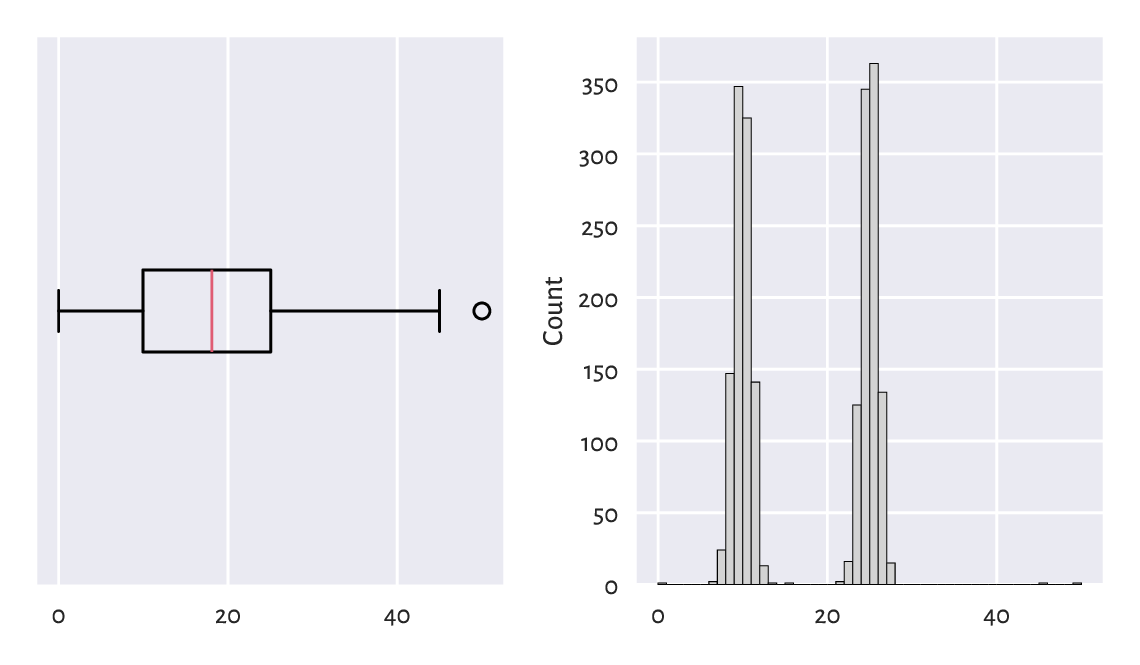Figure 15.2 With box plots, we may fail to detect some outliers

Fixed-radius search techniques that we have discussed in Section 8.4 can be used for estimating the underlying probability density function. Given a data sample $$\boldsymbol{x}=(x_1,\dots,x_n)$$, let us consider1

$\hat{f}_r(z) = \frac{1}{2 r n} \sum_{i=1}^n |B_r(z)|,$

where $$|B_r(z)|$$ denotes the number of observations from $$\boldsymbol{x}$$ whose distance to $$z$$ is not greater than $$r$$, i.e., fall into the interval $$[z-r, z+r]$$.

n = len(x)
r = 1  # radius – feel free to play with different values
import scipy.spatial
t = scipy.spatial.KDTree(x.reshape(-1, 1))
dx = pd.Series(t.query_ball_point(x.reshape(-1, 1), r)).str.len() / (2*r*n)
dx[:6]  # preview
## 0    0.000250
## 1    0.116267
## 2    0.116766
## 3    0.166667
## 4    0.076098
## 5    0.156188
## dtype: float64


Then, points in the sample lying in low-density regions (i.e., all $$x_i$$ such that $$\hat{f}_r(x_i)$$ is small) can be marked for further inspection as potential outliers:

x[dx < 0.001]
## array([ 0.        , 13.57157922, 15.        , 45.        , 50.        ])


See Figure 15.3 for an illustration of $$\hat{f}_r$$. Of course, $$r$$ should be chosen with care – just like the number of bins in a histogram.

sns.histplot(x, binwidth=1, stat="density", color="lightgray")
z = np.linspace(np.min(x)-5, np.max(x)+5, 1001)
dz = pd.Series(t.query_ball_point(z.reshape(-1, 1), r)).str.len() / (2*r*n)
plt.plot(z, dz, label=f"density estimator ($r={r}$)")
plt.show()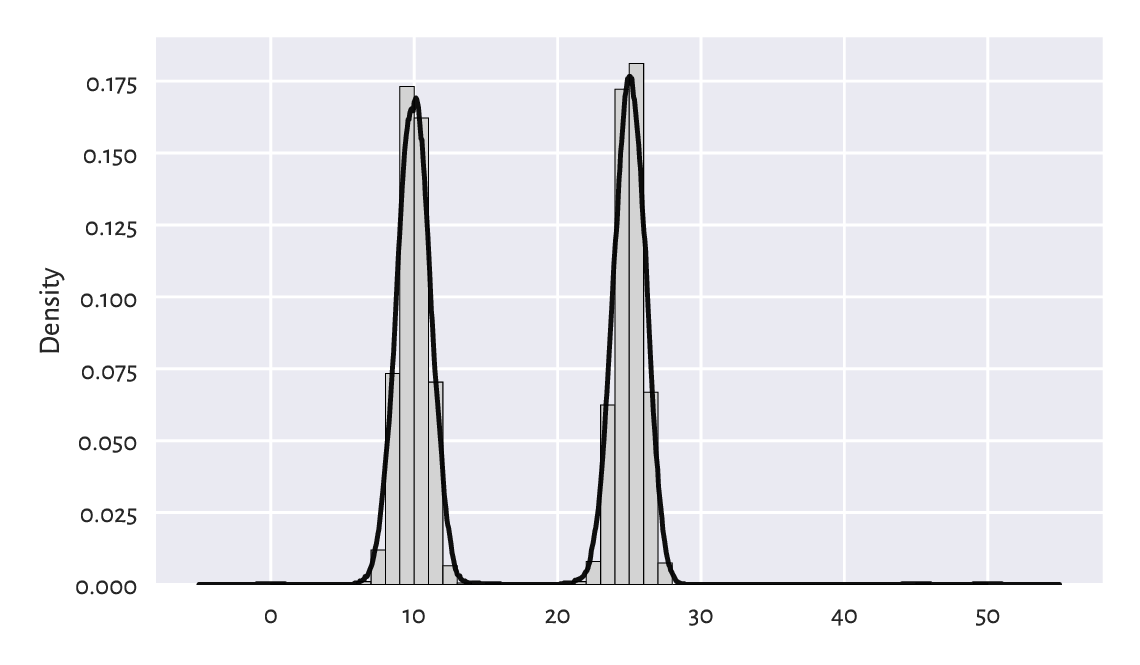Figure 15.3 Density estimation based on fixed-radius search

### 15.4.3. Multidimensional Density Estimation (*)

By far we should have got used to the fact that unidimensional data projections might lead to our losing too much information: some values might seem perfectly fine when they are considered in isolation, but already plotting them in 2D reveals the truth.

Consider the following example dataset and the depiction of the distributions of its two natural projections in Figure 15.4.

X = np.loadtxt("https://raw.githubusercontent.com/gagolews/" +
"teaching_data/master/marek/blobs1.txt", delimiter=",")
plt.figure(figsize=(plt.rcParams["figure.figsize"], )*2)  # width=height
plt.subplot(2, 2, 1)
sns.boxplot(data=X[:, 0], orient="h", color="lightgray")
plt.subplot(2, 2, 2)
sns.histplot(X[:, 0], bins=20, color="lightgray")
plt.title("X[:, 0]")
plt.subplot(2, 2, 3)
sns.boxplot(data=X[:, 1], orient="h", color="lightgray")
plt.subplot(2, 2, 4)
sns.histplot(X[:, 1], bins=20, color="lightgray")
plt.title("X[:, 1]")
plt.show()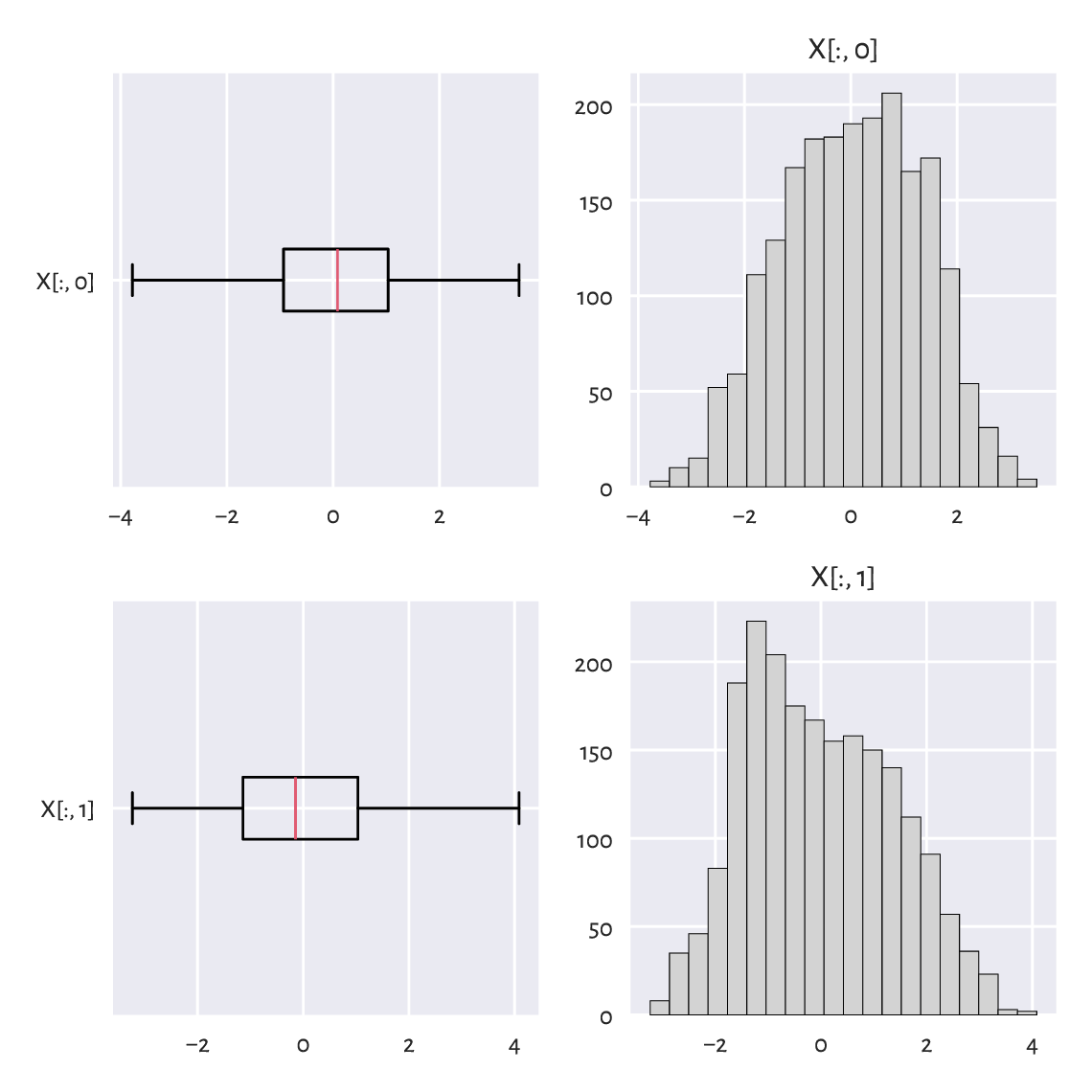Figure 15.4 One-dimensional projections of the blobs1 dataset

There is nothing suspicious here. Or is there?

The scatterplot in Figure 15.5 already reveals that the data consist of two quite well-separable blobs:

plt.plot(X[:, 0], X[:, 1], "o")
plt.axis("equal")
plt.show()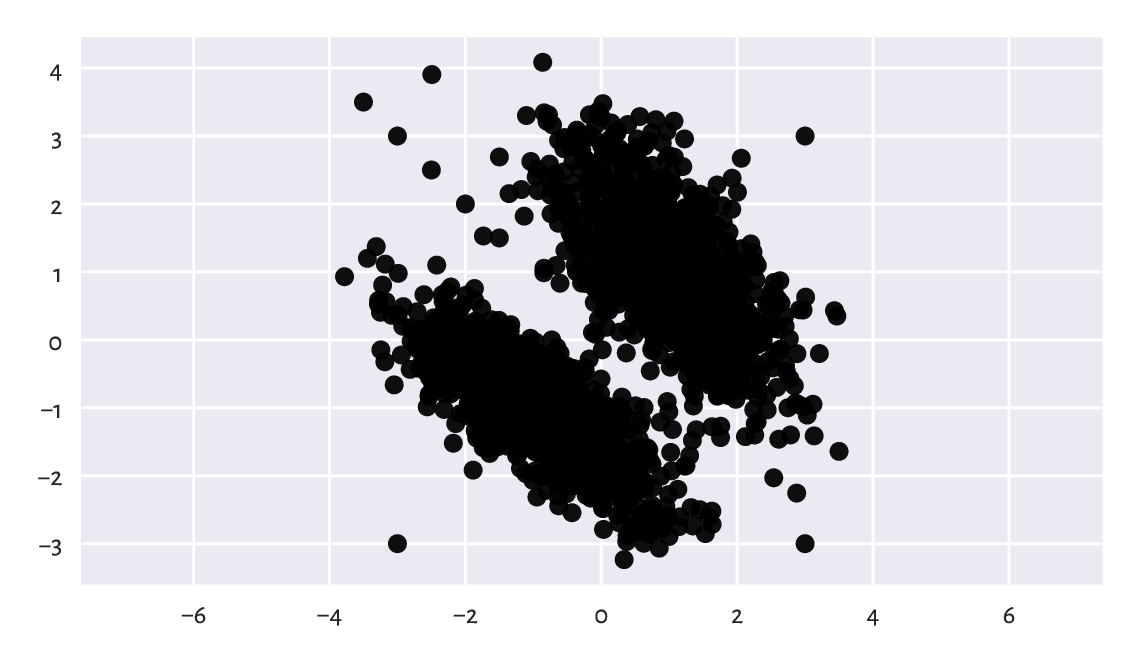Figure 15.5 Scatterplot of the blobs1 dataset

Also, the are a few observations that we might consider outliers. Yours truly included 8 junk points at the very end of the dataset:

X[-8:, :]
## array([[-3. ,  3. ],
##        [ 3. ,  3. ],
##        [ 3. , -3. ],
##        [-3. , -3. ],
##        [-3.5,  3.5],
##        [-2.5,  2.5],
##        [-2. ,  2. ],
##        [-1.5,  1.5]])


Thus, handling multidimensional data requires slightly more sophisticated methods. A quite straightforward approach is to check if there are any points within an observation’s radius of some assumed size $$r>0$$. If that is not the case, we may consider it an outlier. This is a variation on the aforementioned 1-dimensional density estimation approach.

Example 15.11

Consider the following code chunk:

t = scipy.spatial.KDTree(X)
n = t.query_ball_point(X, 0.2)  # r=0.2 (radius)
c = pd.Series(n).str.len().to_numpy()
c[[0, 1, -2, -1]]  # preview
## array([42, 30,  1,  1])


c[i] gives the number of points within X[i, :]’s $$r$$-radius (with respect to the Euclidean distance), including the point itself. Therefore, c[i]==1 denotes a potential outlier, see Figure 15.6 for an illustration.

plt.plot(X[c > 1, 0], X[c > 1, 1], "o", label="normal point")
plt.plot(X[c == 1, 0], X[c == 1, 1], "v", label="outlier")
plt.axis("equal")
plt.legend()
plt.show()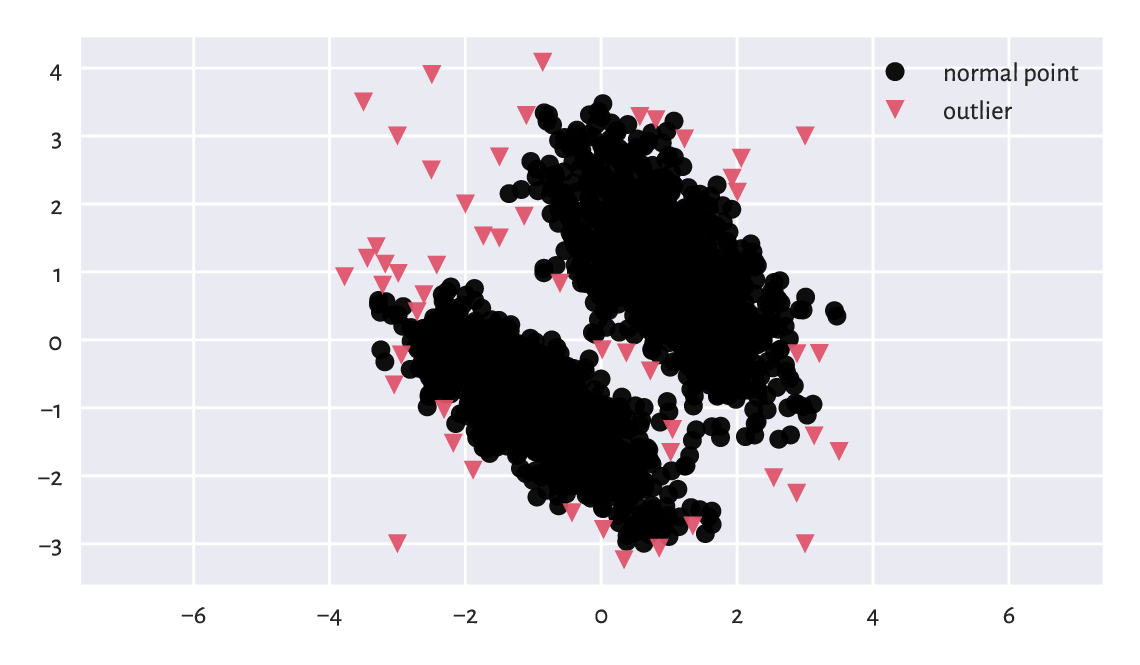Figure 15.6 Outlier detection based on a fixed-radius search for the blobs1 dataset

## 15.5. Exercises

Exercise 15.12

How can missing values be represented in numpy and pandas?

Exercise 15.13

Explain some basic strategies for dealing with missing values in numeric vectors.

Exercise 15.14

Why we should be very explicit about the way we have handled missing and other suspicious data? Is it a good idea to mark as missing or remove completely the observations that we dislike or otherwise deem inappropriate, controversial, dangerous, incompatible with our political views, etc.?

Exercise 15.15

Is replacing missing values with the sample arithmetic mean for income data (as in, e.g., uk_income_simulated_2020.txt`) a sensible strategy?

Exercise 15.16

What is the differences between data missing completely at random, missing at random, and missing not at random (according to Rubin’s [Rub76] classification)?

Exercise 15.17

List some basic strategies for dealing with data that might contain outliers.

1

This is an instance of a kernel density estimator, with the simplest kernel – a rectangular one.